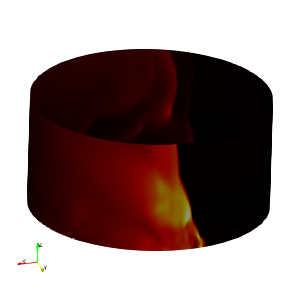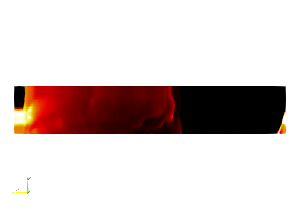# How to unwrap cylinder from a particular location

I am trying to unwrap a cylinder (Figure01) from a particular starting point so that the wave comes in the middle when unwrapped.
But instead, I got this. (Figure02).
I am using this function to unwrap: 0*iHat+(atan(coordsY/coordsX)+(coordsX/abs(coordsX))1.570796326)(coordsX^2+coordsY^2)^(1/2)jHat+coordsZkHat

My question is how do you unwrap from a different starting point?First of all, I recommend changing atan(coordsY/coordsX) to atan2(coordsY,coordsX). The latter is a safer way of computing arctan that avoids divide-by-zero and determines the quadrant correctly. Once you do that, I believe you can remove the (coordsX/abs(coordsX)) part, which I think is trying to address the quadrant problem.

The unwrapping point of atan2 will always be at \pi, but you can rotate your cylinder around the Z axis to change where that \pi position is. A simple math trick to rotate a 2D axis by 90^\circ clockwise is to replace (x,y) \rightarrow (y,-x) (i.e. use atan2(-coordsX, coordsY)). LIkewise 180^\circ is (x,y) -> (-x, -y) and 270^\circ is (x,y) \rightarrow (-y, x).

Or an even easier way that allows for placing the \pi split in an arbitrary position is to apply the Transform before the calculator. Rotate the cylinder around the Z axis.

Thanks Kenneth. It was really helpful.

I got the unwrapped image like this (Figure01). I have also attached another picture (Figure02) showing the settings. Do you know why I am getting gaps in between?

It would help if you share your data, but this might be the same as an issue I ran into when answering your question in that there are polygons that stretched across the plane.

When you converted coordinates to angles, there are some polygons with some points a little less than 180^\circ and others that are a little more than -180^\circ. When drawn as a round cylinder, these points are close to each other. But when the angle is drawn in the x/y plane, these points are on opposite sides of the image. That means the polygons stretch across your entire image and intersect everything. That can create z-buffer fighting that can cause artifacts in the rendering like what you are seeing.

You will have to identify those polygons and remove them. How to do that depends on your data. One way is to use the Cell Size filter to compute the area of your polygons. Then remove the largest polygons, which are probably the ones that are stretched across your data.

Thanks Kenneth.
Do you know, how can I share the data of size 2GB here?

I never used this “Cell Size” filter. Can you please guide how to remove the largest polygons using that? Attached is the Figure showing the properties pane of “Cell Size” filter.

Information about file sharing is in the FAQ: I want to share data here, how can I do that ?

The Cell Size filter itself will not remove the data. It will create a new field that has the area of each polygon in your mesh. Once you have that, you can use something like the Threshold filter to remove the polygons with the largest size.

1 Like